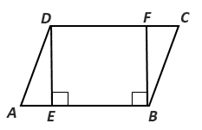Chapter 4.1, Problem 30E### Elementary Geometry for College St...

6th Edition
Daniel C. Alexander + 1 other
ISBN: 9781285195698

#### Solutions

Chapter
Section### Elementary Geometry for College St...

6th Edition
Daniel C. Alexander + 1 other
ISBN: 9781285195698
Textbook Problem
3 views

# In Exercises 27-30, use the definition of a parallelogram to complete each proof.Given: Parallelogram ABCD with D E - ⊥ A B - and F B -   ⊥ A B - Prove: D E - ≅ F B - Plan: First show that DEBF is a parallelogram.To determine

To prove:

DE-FB- in the given figure.

Explanation

Given:

Parallelogram ABCD with DE-AB- and FB- AB-.

Prove: DE-FB-

Corollary:

A parallelogram is a quadrilateral in which both pairs of opposite sides are parallel.

Theorem:

Two parallel line are everywhere equidistance.

From the given parallelogram ABCD, DE-AB- and FB- AB-.

In the parallelogram ABCD, by the corollary AB-DC-

### Still sussing out bartleby?

Check out a sample textbook solution.

See a sample solution

#### The Solution to Your Study Problems

Bartleby provides explanations to thousands of textbook problems written by our experts, many with advanced degrees!

Get Started

#### Find more solutions based on key concepts